离散傅里叶变换DFT与FFT，MATLAB的FFT函数使用（原创）——如何使用fft（）绘制出真正的频谱图像

10 篇文章 2 订阅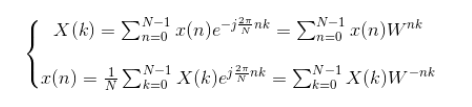OK
1、那么这样FFT第一个步骤就出现了，那就是A/D转换，也就是对连续信号采样。而这里我们就要确定一个参数采样频率Fs，说到采样，大家肯定会想到采样定理，其内容就是当对信号进行以采样频率为Fs>=2fc的采样时，信息不急丢失（fc为原始连续信号的最大截止频率），那么这样第一个参数Fs就这样确定了，其应该满足Fs>=2fc，这时采样周期为Ts=1/Fs。
2、既然确定了采样频率Fs，那么我们就要想信号的时域应该怎么确定，换句话说，我们将采样好的信号给计算机处理时，应该给计算机多少个。这里就出现了第二个参数即序列个数L，好的，在这里由于第一个参数确定的Fs，相应的也是采样周期Ts，所以我们就可以得出时域信号的横坐标时间的变化范围，也就是
t=（0：L-1）Ts

LxTs=L/Fs=1，也就是说其表达的时域t范围为1，那么平常我们的信号最小周期一般都小于1的，要是当信号最小周期大于1时其就是错误的啦，所以这个只是对大部分来说是有用的。

OK

3、于是第三个参数N-DFT的N就出现了，根据上述2情况，我们知道N的提高有利于提高精确的，那么它还有其它约束吗？答案肯定有的，这里就需要些理论知识了：

Fs>=2fc（fc为信号最高截止频率）
LxTs>=T（T为信号的最小周期，若能取LxTs=T则应尽可能取，可以避免频谱泄露）
N>=L（N常常应为2^m，因为FFT运算就是不断模2进行DFT，所以N为2的次方利于提高速度）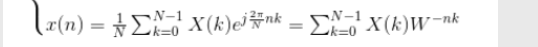Y=fft(x,N);%N一定到大于信号序列x的长度，不过一般等于，决不能小于
%因为若要小于时域就会发生混叠
Y=abs(Y);
Y=Y./N;
%Y=Y.*2./N;Y(1)=Y(1)./2;若考虑将负频率的幅度折算到正频率时应这样处理
%因为变换后有个N的乘积因子的影响，根据DFT公式可知，故消除其影响
f=(0:N-1)*Fs./N;
%频率正常化，因为变换后横坐标是每个点对应的个数，故应转化成实际的频率
%由于频谱是对称的，且周期的故常常只画一半如下
subplot(2,1,1);
plot(f(1:N/2),Y(1:N./2));
%当然也可以画平常我们见到的有对称的如下
f=f-Fs./2;
subplot(212);
plot(f,fftshift(Y));

f=(0:N-1)*Fs./N;这是N-DFT变换后的横轴坐标，也就是真实频率值；
Y=Y./N;这是对DFT变换后幅值处理，也就是要除于N才是真实的幅值
%Y=Y.*2./N;Y(1)=Y(1)./2;而这个是鉴于无真实的负频率，其与正频率对称的原理，把负频率去掉，全变为正频率的幅值处理。

f=f-Fs./2;
subplot(212);
plot(f,fftshift(Y));

OK接下来就是仿真试验啦。

1、首先考虑Fs=512，L=512，N=512，满足上述三个关系吧，
Fs=512>=2fc；LxTs=1s>=T（也是10T，故没频率泄露）；N=512>=L=512；那么我们看下仿真结果是否对得到：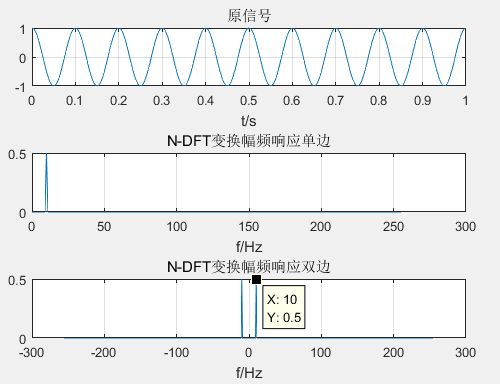Fs=512;%采样频率
Ts=1/Fs;%采样周期
N=512;%N—DFT
L=512;%原信号序列长度
t=(0:L-1).*Ts;%时域自变量
x=cos(10*2*pi*t);%原信号
subplot(311);
plot(t,x);
title("原信号")
xlabel("t/s")
grid on
Y=fft(x,N);%fft变换
Y=abs(Y)./N;%实际幅值变换
f=(0:N-1)*Fs./N;%实际频率变换
subplot(312);
plot(f(1:N/2),Y(1:N./2));
title("N-DFT变换幅频响应单边")
xlabel("f/Hz")
grid on
f=f-Fs./2;%移位
subplot(313);
plot(f,fftshift(Y));%移位
title("N-DFT变换幅频响应双边")
xlabel("f/Hz")
grid on

2、那么我们再来考虑Fs=512，L=128，N=128，满足上述三个关系吧，
Fs=512>=2fc；LxTs=0.15s>=T（不是T的整数倍，故有频率泄露）；N=128>=L=128；那么我们看下仿真结果是否对得到（这次频谱用stem绘制更明显点）：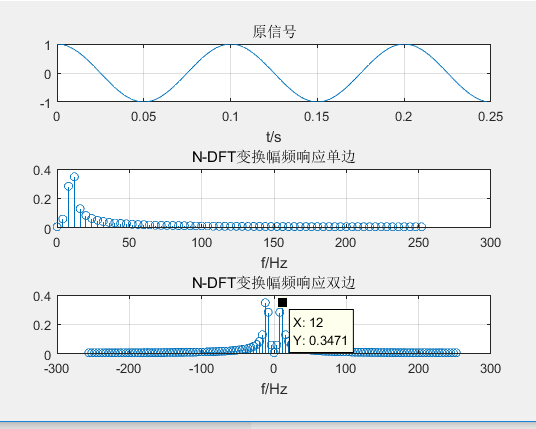Fs=512;%采样频率
Ts=1/Fs;%采样周期
N=128;%N—DFT
L=128;%原信号序列长度
t=(0:L-1).*Ts;%时域自变量
x=cos(10*2*pi*t);%原信号
subplot(311);
plot(t,x);
title("原信号")
xlabel("t/s")
grid on
Y=fft(x,N);%fft变换
Y=abs(Y)./N;%实际幅值变换
f=(0:N-1)*Fs./N;%实际频率变换
subplot(312);
stem(f(1:N/2),Y(1:N./2));
title("N-DFT变换幅频响应单边")
xlabel("f/Hz")
grid on
f=f-Fs./2;%移位
subplot(313);
stem(f,fftshift(Y));%移位
title("N-DFT变换幅频响应双边")
xlabel("f/Hz")
grid on

3、那么我们再来看下增大N点是否可以减小频谱泄露的影响，考虑Fs=512，L=128，N=1024，满足上述三个关系吧，
Fs=512>=2fc；LxTs=0.15s>=T（不是T的整数倍，故有频率泄露）；N=1024>=L=128；那么我们看下仿真结果是否对得到（这次频谱用stem绘制更明显点）：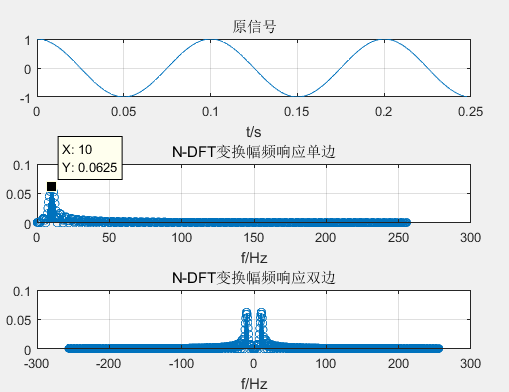Fs=512;%采样频率
Ts=1/Fs;%采样周期
N=1024;%N—DFT
L=128;%原信号序列长度
t=(0:L-1).*Ts;%时域自变量
x=cos(10*2*pi*t);%原信号
subplot(311);
plot(t,x);
title("原信号")
xlabel("t/s")
grid on
Y=fft(x,N);%fft变换
Y=abs(Y)./N;%实际幅值变换
f=(0:N-1)*Fs./N;%实际频率变换
subplot(312);
stem(f(1:N/2),Y(1:N./2));
title("N-DFT变换幅频响应单边")
xlabel("f/Hz")
grid on
f=f-Fs./2;%移位
subplot(313);
stem(f,fftshift(Y));%移位
title("N-DFT变换幅频响应双边")
xlabel("f/Hz")
grid on

4、最后看下它可不可ifft（）变回去：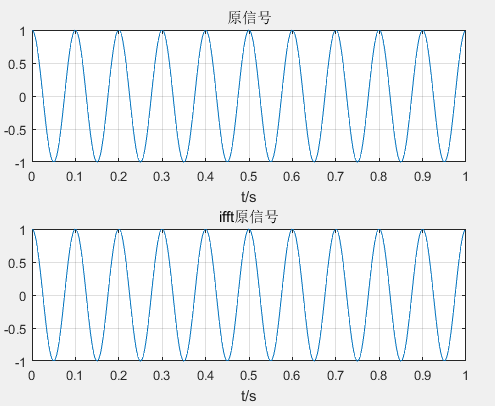figure
subplot(211)
plot(t,x)
title("原信号")
xlabel("t/s")
grid on
Y=fft(x);
xx=ifft(Y);
subplot(212)
plot(t,xx)
title("ifft原信号")
xlabel("t/s")
grid on

07-11
12-1617万+

10-31
11-125729
10-285万+
06-231万+
05-259万+
09-073万+
06-033万+
04-08718
11-2074
03-19186
11-20270
12-06
06-122637
11-19
08-198462
03-20767
03-19192
05-263007¥2 ¥4 ¥6 ¥10 ¥20余额支付 (余额：-- )扫码支付获取中扫码支付点击重新获取扫码支付1.余额是钱包充值的虚拟货币，按照1:1的比例进行支付金额的抵扣。
2.余额无法直接购买下载，可以购买VIP、C币套餐、付费专栏及课程。余额充值